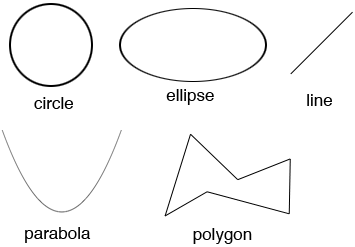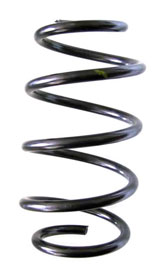SEARCH HOMEMath Central Quandaries & QueriesQuestion from ann, a parent: what does planar curve mean in your definition of a cone?Hi Ann,

A planar curve is one that lies in a plane. Some examples areTo be the base of a cone the planar curve must be closed. In the plane, a closed curve is a curve with no endpoints and which completely encloses an area. (See Wolfram Mathworld.) Thus the line and parabola above can not be the base of a cone.

An example of a curve that is not planar is the curve formed by a coil spring.PennyMath Central is supported by the University of Regina and the Imperial Oil Foundation.